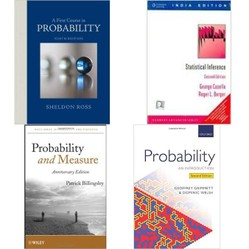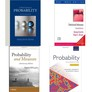# Probability Theory Books - Best Textbooks for Probability

by cazort

## Here I review textbooks in probability theory, from the introductory level through more advanced texts. I have chosen only books I consider to be the best of the best.

Probability theory is a subject that requires considerable math, but can be both fun and intuitive. It is often easily understood in terms of games of chance, which is why I chose to display a picture of dice on the left. It is an immensely useful subject, with applications ranging from statistics and data analysis, to business, to gambling and games.

I start by explaining the differences between the different approaches taken by different textbooks in the subject of probability theory. Skip below if you wish to get straight to the reviews!

I outline pros and cons for each book.

### Different Types of Probability Textbooks

##### Your purposes behind learning this subject will determine which book is best for you!

Probability is such an important subject that it is both an interesting subject in its own right, and a key theoretical underpinning of many subjects, including stochastic processes and statistics. Because the subject can be taken in different directions, I am grouping the probability textbooks I review into the following categories:

• Pure probability textbooks - These books introduce basic probability theory as a subject in its own right. These books include the Grimmett and Welsh, and Sheldon Ross' A First Course book.
• Stochastic processes - A major application of probability theory, as well as a field of study in its own right, is the theory of stochastic processes, processes involving randomness or chance. Some elementary textbooks are written with an eye towards this material; Sheldon Ross' book Probability Models is an example of such a text.
• Probability and statistics - Probability theory is the mathematical underpinning of mathematical statistics and theories of statistical inference, and statistics represents another direction that probability theory can be taken in. On this page, I include the Fisz text, which is a predominately pure probability book that moves in the direction of statistics, as well as a book by Casella and Berger that is predominately a mathematical statistics textbook, but which has an outstanding exposition of probability theory.
• Measure-theoretic approaches - These books use mathematically advanced theories of measure and integration (from the area of real analysis) to provide a mathematically rigorous underpinning to the theory of probability and statistics. These books are not typically necessary unless you are doing Ph.D.-level work. The Billingsley book is an example of a book taking a measure-theoretic approach to this subject.

Which of these books is best for you will depend on why you want to learn probability. I personally recommend getting multiple books, because I think it is worthwhile to at least see the applications of probability, especially in statistics, and I also think that, even if you don't directly work in stochastic processes, the theory of stochastic processes can help you understand certain probability distributions in a deeper and more intuitive way.

### Probability: An Introduction by Grimmett and Welsh

##### A little-known, concise, but outstanding text for mathy people: two editions shown.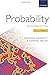Probability: An IntroductionProbability is an area of mathematics of tremendous contemporary importance across all aspects of human endeavour. This book is a compact account of the basic features of probab...View on Amazon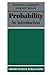Probability: An Introduction (Oxford Science Publications)This new undergraduate text offers a concise introduction to probability and random processes. Exercises and problems range from simple to difficult, and the overall treatment, ...View on Amazon

Probability: An Introduction, by Geoffrey Grimmett and Dominic Welsh, is a little-known and much overlooked textbook, and is my favorite introduction to the topic. I recommend it for undergraduate math majors and other people who have a more mathy orientation and like denser books.

Pros: This volume is compact and inexpensive. The authors do a very good job of helping develop the reader's intuition. I also find this book makes a good reference. Covers a great deal of material relative to its small size, getting into some material on stochastic processes.

Cons: There are relatively few examples, and I think more would be beneficial. The text's conciseness, dense nature, and quicker pace may make it a little tough for students who do not have prior experience in abstract math courses.

### Probability Theory and Mathematical Statistics by Marek Fisz

##### An old, out-of-print textbook with an excellent take on probability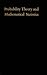Probability Theory and Mathematical StatisticsView on Amazon

Probability Theory and Mathematical Statistics by Marek Fisz, is an older textbook that I like very much. In spite of the title, this book is primarily about probability and only gets into a little bit of mathematical statistics at the very end, which is why I am reviewing it here and not on my page on statistics.

Pros: This book occupies a pleasant middle ground between the more theoretical math-oriented books, and the basic intro-level textbooks. The fact that the theory is designed with an eye towards mathematical statistics, and that the book covers some of this material, is also refreshing, as many probability textbooks completely ignore statistics, which is arguably the most important application of the theory.

Cons: Sometimes old-fashioned presentation. Out-of-print and may be difficult to obtain. Check Amazon marketplace and Half.com for used copies.

### A First Course in Probability by Sheldon Ross

##### An introductory text that has been through too many editions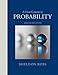A First Course in Probability (9th Edition)A First Course in Probability, Ninth Edition, features clear and intuitive explanations of the mathematics of probability theory, outstanding problem sets, and a variety of dive...View on Amazon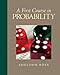A First Course in Probability (8th Edition)A First Course in Probability, Eighth Edition, features clear and intuitive explanations of the mathematics of probability theory, outstanding problem sets, and a variety of div...View on Amazon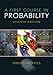First Course in Probability, A (7th Edition)This introduction presents the mathematical theory of probability for readers in the fields of engineering and the sciences who possess knowledge of elementary calculus. Present...View on Amazon

Sheldon Ross' A First Course in Probability is a textbook that I have mixed feelings about. On its own, I would not recommend it, but it may be useful as a supplement. If you buy this book, I strongly recommend buying an older edition. With some effort, you can find older editions, used, for under \$10. This book has been through 8 editions but there have been few positive improvements since around the 6th.

Pros: Written at a more accessible level, requiring a little less math knowledge than some of the other books reviewed here. Extremely rich examples. I think the examples are the best part of this book, and this alone might make this a book worth buying.

Cons: Completely ignores the fact that the field of statistics exists and does not even mention applications to statistics. Lacks explanatory prose or discussion of the theory. In spite of its introductory level, may require either a good teacher or a supplemental text to fill in some gaps. Newer editions have fattened the book with examples, but have been carried out sloppily, introducing new typos and errors. This book presents the worst example of "edition creep" of a math book that I've ever seen. Note: the book does become more concise in earlier editions, so people wanting more examples may wish to buy 6th edition or later.

### Introduction to Probability Models by Sheldon Ross

##### Emphasizing stochastic processes, faster-paced but similar to the other Ross text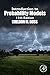Introduction to Probability Models, Eleventh EditionSheldon Ross's classic bestseller, Introduction to Probability Models, has been used extensively by professionals and as the primary text for a first undergraduate course in app...View on Amazon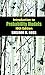Introduction to Probability Models, Tenth EditionIntroduction to Probability Models, Tenth Edition, provides an introduction to elementary probability theory and stochastic processes. There are two approaches to the study of p...View on Amazon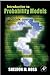Introduction to Probability Models, Ninth EditionRoss's classic bestseller, Introduction to Probability Models, has been used extensively by professionals and as the primary text for a first undergraduate course in applied pro...View on Amazon

Introduction to Probability Models moves at a slightly faster pace, especially in early chapters, and covers more material. Unfortunately, it suffers from the same problems of edition creep that the more introductory textbook does, so I recommend buying an older edition (no later than 9th) to save money.

Pros: Very good at developing intuition for probabilistic reasoning. Rich in examples.

Cons: Faster pace of introductory material may make this book a little less accessible than Sheldon Ross' other book. This book has a major omission, in my opinion, in that it does not discuss or even mention any applications of the probability models here to mathematical statistics or statistical inference.

### Casella and Berger: Statistical Inference

##### A statistics book with an exceptionally lucid presentation of probability theory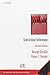Statistical InferenceThis text provides information on topics such as ancillarity, invariance, Bayesian methods, pivots, Stein estimation, errors in variables and inequalities. The authors discuss b...View on Amazon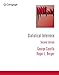Statistical InferenceThis book builds theoretical statistics from the first principles of probability theory. Starting from the basics of probability, the authors develop the theory of statistical i...View on Amazon

Statistical Inference is primarily a book on mathematical statistics. It is not my favorite book on statistics, but I am including it here for two reasons: I think that the book's exposition of probability theory at the beginning is one of its strongest suits, and the fact that the book develops the subject with an eye towards its use in statistics is a strength that complements one of the most common weaknesses of introductory textbooks, including many of the ones I recommended above.

Pros: Excellent exposition of probability theory, developed with an eye towards its use in statistics. Abundant exercises in problem sets. Clean use of mathematical notation, and thorough in terms of exploring the manipulations of mathematical formulas that typically arise in statistics.

Cons: At times this book tends towards tedious manipulations. In spite of its name, not a great text for a statistician; weak in many areas of statistics (especially data analysis).  Also contains almost no discussion of stochastic processes.

### Probability and Measure by Patrick Billingsley

##### A classic text on measure-theoretic probability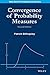Convergence Of Probability Measures 2Ed (Pb 2014)View on Amazon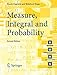Measure, Integral and ProbabilityView on Amazon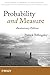Probability and MeasureView on Amazon

Probability and Measure is a classic, "old-school" text in measure-theoretic probability; it's the sort of text that an older professor would be likely to have on their shelf and/or recommend.  Although I normally shy away from these sorts of books, I think this is one older book worth looking at.

Pros: This book does an outstanding example of introducing the rigorous theory of measure and integration in such a way that integrates it with the applications in the theories of probability and stochastic processes for which it is most useful. It takes a painfully abstract subject and makes it surprisingly captivating.

Cons: As clear as this book is, it's covering a very tough subject. An overwhelming majority of students will neither need this book's material, nor be able to easily understand it. I recommend strong prior exposure to mathematical analysis (advanced calculus), and ideally, some exposure to topology and other abstract math that heavily uses set theory.

### Free Online Probability Textbooks

##### Every resource in this section is available online, free of charge!

Probability is one subject within mathematics for which there are outstanding free online resources available. Here I have selected three online textbooks, which are the most useful resources I know about in book-like format (two of them are actually published in hard-copy books as well).

There are other online resources that can help you learn probability, but I have chosen to focus on these because they are books or set up like books. I personally find the book format helpful for learning.

### More of my Math Book Reviews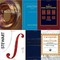Reviews and recommendations of textbooks in Calc I, II, and III/Multivariable, as well as supplemental books for self-study or enrichment.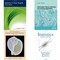Reviews of introductory textbooks in statistics, from more general-audience books to mathy books for advanced or graduate students.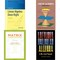Recommendations and reviews of textbooks for linear algebra at both undergraduate (college) and graduate levels.
Updated: 04/02/2015, cazort
Thank you! Would you like to post a comment now?
2

### Questions? Comments? Feedback?

Only logged-in users are allowed to comment. Login

#### You might also like

Calculus Books - Best Textbooks for Calculus

Reviews and recommendations of textbooks in Calc I, II, and III/Multivariable...

Linear Algebra Textbook Reviews

Recommendations and reviews of textbooks for linear algebra at both undergrad...

Disclosure: This page generates income for authors based on affiliate relationships with our partners, including Amazon, Google and others.